1. /
2. CBSE
3. /
4. Class 11
5. /
6. Correlation class 11 Notes...

# Correlation class 11 Notes Economics### myCBSEguide App

Download the app to get CBSE Sample Papers 2023-24, NCERT Solutions (Revised), Most Important Questions, Previous Year Question Bank, Mock Tests, and Detailed Notes.

CBSE Economics Chapter 7 Correlation class 11 Notes Economics in PDF are available for free download in myCBSEguide mobile app. The best app for CBSE students now provides Correlation class 11 Notes Economics latest chapter wise notes for quick preparation of CBSE exams and school based annual examinations. Class 11 Economics notes on Chapter 7 Correlation class 11 Notes Economics are also available for download in CBSE Guide website.

## CBSE Guide Correlation class 11 Notes

CBSE guide notes are the comprehensive notes which covers the latest syllabus of CBSE and NCERT. It includes all the topics given in NCERT class 11 Economics text book. Users can download CBSE guide quick revision notes from myCBSEguide mobile app and my CBSE guide website.

## Correlation class 11 Notes Economics

Download CBSE class 11th revision notes for Chapter 7 Correlation class 11 Notes Economics in PDF format for free. Download revision notes for Correlation class 11 Notes Economics and score high in exams. These are the Correlation class 11 Notes Economics prepared by team of expert teachers. The revision notes help you revise the whole chapter in minutes. Revising notes in exam days is on of the best tips recommended by teachers during exam days.

CBSE Class 11 Economics
Revision Notes
Chapter – 7
Correlation class 11 Notes Economics

Points to remember
Meaning of correlation:
Correlation is a statistical tool which studies the relationship between two variables e.g. change in price leads to change in quantity demanded.
Correlation studies and measures the direction and intensity of relationship among variables. It measures co-variation not causation. It does not imply cause and effect relation.

Type of Correlation
Correlation is classified into positive and negative correlation. The correlation is said to be positive when the variables move together in the same direction. e.g. sale of lce cream and temperature move in same direction.
The correlation is said to be negative when the variables move in opposite direction. e.g. When you spend more time in studying chances of your failure decline.

Examples of positive correlation are:
1. Price and supply of a commodity.
2. Increase in Height and Weight.
3. Age of husband and age of wife.
4. The family income and expenditure on luxury items.

Examples of negative correlation are:
1. Sale of woollen garments and day temperature.
2. Price and Demand of a commodity.
3. Yield of crops and price.

Degree of Correlation:

 Degree Positive Negative Perfect +1 -1 High Between +0.75 and +1 Between -0.75 and -1 Moderate Between +0.25 and +0.75 Between -0.25 and -0.75 Low Between 0 and +0.25 Between 0 and -0.25 Zero 0 0

Methods of estimating correlation:
(a) Scatter diagram
(b) Karl person’s coefficient of correlation.
(c) Spearman’s rank correlation.

Scatter diagram offers a graphic expression of the direction and degree of correlation. To construct a scatter diagram, x variables taken on X-axis and y variable is taken on Y-axis. The cluster of points, plotted is referred to as a scatter diagram. In this, the degree of closeness of scatter points and their overall direction enables us to examine the relationship.
Karl person’s coefficient of correlation is a quantitative method of calculating correlation. It gives a precise numerical value of the degree of linear relationship between two variables.
Karl person’s coefficient of correlation is also known as product moment correlation.
Formula: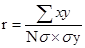Here,
r = Coefficient of correlation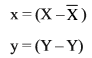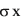= Standard deviation of X-series.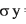= Standard deviation of Y-series.
N = Number of observations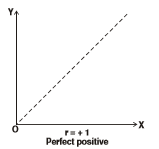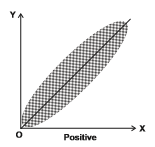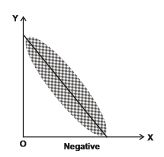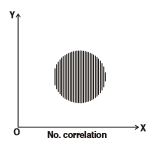Karl Person’s coefficient of correlation is calculated by following methods:

(a) Actual mean method: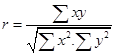Here,
r = Coeff. Of correlation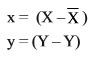(b) Assumed Mean method: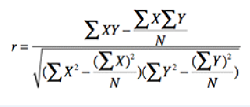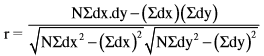Here,
dx = Deviations of x-series from assumed mean = (X – A)
dy = Deviation of Y-series from assumed mean = (Y – A)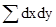= Sum of multiple of dx and dy.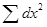= Sum of the square of dx.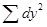= Sum of the square of dy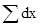= Sum of the deviation of x-series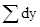= Sum of the deviation of Y-series
N = Number of pairs of observations
When value of the variables are large, we use step deviation method to reduce the burden of calculation.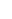(c) Step deviation method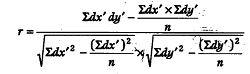Here,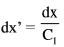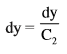dx = deviation of X-series from assumed mean = (X-A)
dy = deviation of Y-series from assumed mean = (Y-A)= Sum of multiple of dx and dy.= Sum of the square of dx.= Sum of the square of dy= Sum of the deviation of x-series= Sum of the deviation of Y-series
N = Number of pairs of observations
C1 is common factor for series -x
C2 is common factor for series -y

Properties of correlation coefficient(r)
(i) Correlation coefficient (r) has no unit.
(ii) A negative value of r indicates an inverse relation.
(iii) If r is positive then two variables move in the same direction.
(iv) The value of r lies between minus – 1 and +1, i.e.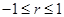(v) If r is zero, the two variables are uncorrelated.
(vi) If r = + 1 or r = – 1, the correlation is perfect.
(vii) A high value of r indicates strong linear relationship and a low value or indicates a weak linear relationship.
(viii) The value of r is unaffected by the change of origin and change of scale.

Given two variables x and y let us define two new variables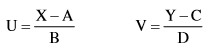Here A and C are assumed means of X and V respectively. B and D are common factors. They rxy = ruv.

Spearman’s rank correlation method is used to calculate coefficient of correlation of qualitative variables such as beauty, bravery, wisdom, ability virtue etc. It was developed by British Psychologist C.E. spearman.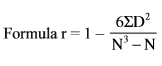Here,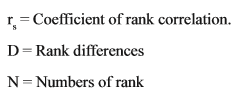When ranks are repeated the formula is: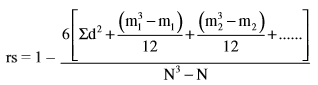Where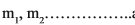are number of repetitions of ranks.

## Correlation class 11 Notes

• CBSE Revision notes for Class 11 Economics PDF
• CBSE Revision notes Class 11 Economics – CBSE
• CBSE Revisions notes and Key Points Class 11 Economics
• Summary of the NCERT books all chapters in Economics class 11
• Short notes for CBSE class 11th Economics
• Key notes and chapter summary of Economics class 11
• Quick revision notes for CBSE exams

## CBSE Class-11 Revision Notes and Key Points

Correlation class 11 Notes Economics. CBSE quick revision note for class-11 Mathematics, Physics, Chemistry, Biology and other subject are very helpful to revise the whole syllabus during exam days. The revision notes covers all important formulas and concepts given in the chapter. Even if you wish to have an overview of a chapter, quick revision notes are here to do if for you. These notes will certainly save your time during stressful exam days.

To download Correlation class 11 Notes, sample paper for class 11 Chemistry, Physics, Biology, History, Political Science, Economics, Geography, Computer Science, Home Science, Accountancy, Business Studies and Home Science; do check myCBSEguide app or website. myCBSEguide provides sample papers with solution, test papers for chapter-wise practice, NCERT solutions, NCERT Exemplar solutions, quick revision notes for ready reference, CBSE guess papers and CBSE important question papers. Sample Paper all are made available through the best app for CBSE students and myCBSEguide website.Test Generator

Create question paper PDF and online tests with your own name & logo in minutes.myCBSEguide

Question Bank, Mock Tests, Exam Papers, NCERT Solutions, Sample Papers, Notes